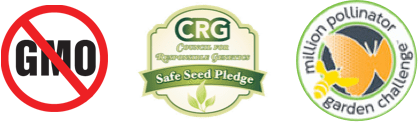# Flowers for a Spring Pollinator Garden

Plant these early bloomers for abundant early to mid season blossoms that feed and nurture many kinds of beneficial insects.

-----TOP CONTENT ABOVE HERE-----

LEFT COLUMN CONTENT BELOW HERE

LEFT COLUMN CONTENT ABOVE HERE

MIDDLE COLUMN CONTENT BELOW HERE

MIDDLE COLUMN CONTENT ABOVE HERE

RIGHT COLUMN CONTENT BELOW HERE

RIGHT COLUMN CONTENT ABOVE HERE

LEFT COLUMN CONTENT BELOW HERE

LEFT COLUMN CONTENT ABOVE HERE

MIDDLE COLUMN CONTENT BELOW HERE

MIDDLE COLUMN CONTENT ABOVE HERE

RIGHT COLUMN CONTENT BELOW HERE

RIGHT COLUMN CONTENT ABOVE HERE

LEFT COLUMN CONTENT BELOW HERE

LEFT COLUMN CONTENT ABOVE HERE

MIDDLE COLUMN CONTENT BELOW HERE

MIDDLE COLUMN CONTENT ABOVE HERE

RIGHT COLUMN CONTENT BELOW HERE

RIGHT COLUMN CONTENT ABOVE HERE

LEFT COLUMN CONTENT BELOW HERE

LEFT COLUMN CONTENT ABOVE HERE

MIDDLE COLUMN CONTENT BELOW HERE

MIDDLE COLUMN CONTENT ABOVE HERE

LEFT COLUMN CONTENT BELOW HERE

LEFT COLUMN CONTENT ABOVE HERE

MIDDLE COLUMN CONTENT BELOW HERE

MIDDLE COLUMN CONTENT ABOVE HERE

RIGHT COLUMN CONTENT BELOW HERE

RIGHT COLUMN CONTENT ABOVE HERE

LEFT COLUMN CONTENT BELOW HERE

LEFT COLUMN CONTENT ABOVE HERE

MIDDLE COLUMN CONTENT BELOW HERE

MIDDLE COLUMN CONTENT ABOVE HERE

RIGHT COLUMN CONTENT BELOW HERE

RIGHT COLUMN CONTENT ABOVE HERE